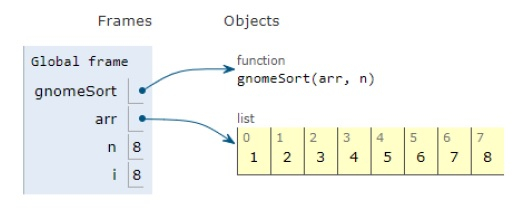# Python Program for Gnome Sort

PythonServer Side ProgrammingProgramming

Problem statement − We are given an array, we need to sort it using gnome sort.

## Algorithm

1. Firstly we traverse the array from left to right.
2. Now,if the current element is larger or equal to the previous element then we traverse one step ahead
3. otherwise,if the current element is smaller than the previous element then swap these two elements and traverse one step back.
4. Repeat steps given above till we reach the end of the array

Now let’s observe the solution in the implementation below −

## Example

Live Demo

def gnomeSort( arr, n):
index = 0
while index < n:
if index == 0:
index = index + 1
if arr[index] >= arr[index - 1]:
index = index + 1
else:
arr[index], arr[index-1] = arr[index-1], arr[index]
index = index - 1
return arr
# main
arr = [1,4,2,3,6,5,8,7]
n = len(arr)
arr = gnomeSort(arr, n)
print ("Sorted sequence is:")
for i in arr:
print (i,end=" ")

## Output

Sorted sequence is:
1 2 3 4 5 6 7 8All the variables are declared in the local scope and their references are seen in the figure above.

## Conclusion

In this article, we have learned about how we can make a Python Program for Gnome Sort